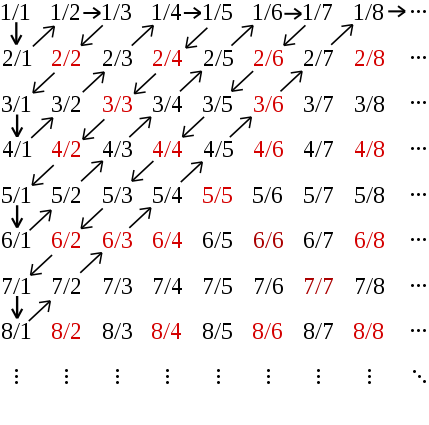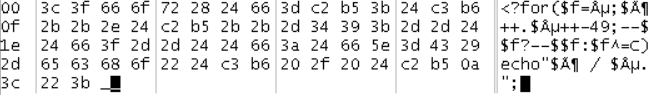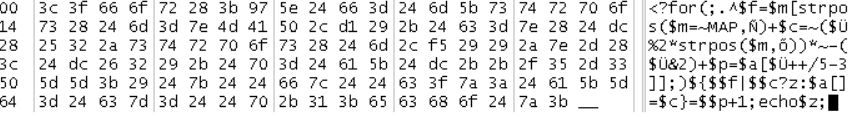Information security, life and stuffCode golfing is about creating the shortest code, in bytes, to solve a given problem, in a specific language or free of choice. It would be the opposite of this.

Examples of on-going challenges:

This guide was originally written by JWvdVeer and Wim for phpGolf.org. I have done some editing and fine tuning before releasing it again here. Although this guide is written for PHP version 5.3.3, the same principles still applies today, but does not include things like e.g. short array syntax. This is not an exhausting list of all tricks, but these might be the most important ones. The examples in the guide are not meant as the optimal solution for the given problem, but to show off the trick in question. The code written in the guide expects that error reporting is set to E_ALL & ~E_NOTICE.

I have also added some of the most impressive submissions on phpGolf.org down below to take inspiration from.

## General Tips

• PHP behaves in a consistent way, so you can always predict the outcome of the code. Sometimes you might even exploit known odd behavior of PHP.
• Know the environment your code will run in. Most challenges have error_reporting E_ALL & ~E_NOTICE. So notices about functions being deprecated or undefined array indexes are accepted.
• Use Google. Some challenges are copies of other challenges on the Internet. Or they are much the same. So you can get some inspiration of sometimes complete challenges.
• Most of the time the less variables you use will result in a smaller solution in bytes. Ask yourself these questions: Do I really need them, or might the value of this variable also be derived from any other variable? Can I combine two values into one in order to save even 1 byte?

## Numbers

Instead of writing 1000 you can write 1e3. 1000000 would be 1e6 etc.

## Strings

A lot of space can be saved when dealing with strings.

### Substrings

Substrings can be accessed like arrays, which means that you can do this:

### String inversion

Many strings doesn’t need to be quoted when notices are turned off (~E_NOTICE), which means that that the following will work, thus saving 2 bytes:

This will however not work:

If you have a string with whitespace or characters that needs to be quoted, you can invert the string.

This code prints a newline, using 8 bytes:

This does the same thing using 7 bytes:

And finally this does also the same, but using 6 bytes:

Regular expressions are a good example of a kind of strings you can save bytes on using this trick.

You could save 2 bytes doing this:

Make sure to set your text editor to latin1 (ISO-8859-1 or Windows-1252) instead of UTF8 otherwise you will save those inverted bytes as multi-bytes which will do the opposite of what we are trying to do here.

Sometimes you can also invert the input to shorten your overall code.

A list of useful inverted characters:

``````* Tab (char 9) -> ~ö
* Line-feed (char 10) -> ~õ
* Space (char 32) -> ~ß
* '!' (char 33) -> ~Þ
* '"' (char 34) -> ~Ý
* '#' (char 35) -> ~Ü
* '\$' (char 36) -> ~Û
* '%' (char 37) -> ~Ú
* '&' (char 38) -> ~Ù
* ''' (char 39) -> ~Ø
* '(' (char 40) -> ~×
* ')' (char 41) -> ~Ö
* '*' (char 42) -> ~Õ
* '+' (char 43) -> ~Ô
* ',' (char 44) -> ~Ó
* '-' (char 45) -> ~Ò
* '.' (char 46) -> ~Ñ
* '/' (char 47) -> ~Ð
* ':' (char 58) -> ~Å
* ';' (char 59) -> ~Ä
* '<' (char 60) -> ~Ã
* '=' (char 61) -> ~Â
* '>' (char 62) -> ~Á
* '?' (char 63) -> ~À
* '[' (char 91) -> ~¤
* '\' (char 92) -> ~£
* ']' (char 93) -> ~¢
* '^' (char 94) -> ~¡
* '_' (char 95) -> ~  (char 160, the fact you are not able to see whitespace doesn't mean that PHP treat is as whitespace!)
``````

## Arrays

Unless you’re performing an array manipulation, most references to an array index \$a[\$i] can be replaced with simply \$\$i. This is even true if the index is an integer, as integers are valid variable names in PHP (although literals will require brackets, e.g. \${0}).

Example of populating an “array”:

``````<?for(\$i=0; \$i<10; \$i++) \$\$i = \$i*2;
``````

## Control Structures

### Braces

Know where you need brackets and where you don’t. If a statement is only one line, you don’t need brackets. Compare these two examples that print chars below 1000 that have a “9” in it.

### Multiple statements

Often it happens that you have multiple statements inside an if statement:

You can rewrite this as:

Or even better:

Or as optimal as we know it:

This works because print always returns 1.

### Loops

Never use while loops. For loops are always at least as short as a while loop, and most of the time shorter. The following code is a not very optimized version of rot13.

Try to use as few control structures as possible. Multiple loops can often be folded into a single loop.

### Ifs

Try to avoid the use of the traditional if-structure. Most times the same action can be done by using the ternary operator. The next three code-snippets are exactly the same:

The following code prints the values of pow(3,n), n<10, starting with n=0:

If you do have only an if (and no else), try to negate the condition. Since the middle part of the of ternary operator might be left out.

So:

Equals (Since PHP 5.3>):

Even equals:

Since the associativity of this operator is left, nested ternary-operators should be preferable done in the true-action, since you otherwise have to use parentheses.

Same code, but false-based:

Compare the examples below that both print all primes below 1000.

The whole if-structure can here be replaced with a ternary operator. Also try to avoid the need of keywords like ‘break’ and ‘continue’, since they need a lot of bytes, while it even might be done using a variable, that perhaps even might be used for other purposes.

Rewritten without if and continue, although this is far from the optimal solution:

## Functions

You should (almost) never write your own functions. In most cases it is unnecessary and it costs a lot of bytes. Some built-in functions in PHP should never be used. These are some examples with a better equivalent to the right.

In some cases, print might be useful since it can be used as a function while echo can’t:

• lcfirst -> `\$a|' '` or `\$a|~ß`
• strtoupper(\$a) -> if \$a is only one char, you can use: `\$a&ß` or `\$a&'ß'`
• ucfirst: see strtoupper
• echo sprintf(…) -> printf(…)
• str_replace -> consider whether strtr can be used
• array_unique -> Most times this function is useless. If something has to be unique, you can do it in many different ways dependent on context.

All three examples below shows all unique letters in the string in capitals:

• sizeof -> most times unnecessary, if needed use count.
• count -> most times unnecessary. See examples below:
• floor -> Often can done with (int)\$a (If you only want to do echo floor(\$a); you might consider printf(‘%u’,\$a);)
• preg_replace and preg_filter does have an e-flag, which means that the replacement is being executed as shown in the example below.

Both solutions below are written by JWvdVeer for the PIG-latin golf challenge:

The second solution is much shorter than the first. It even can handle strings with punctuation.

## Operators

### Precedence

Know the precedence of operators. A table with information about the precedence can be found at: http://php.net/manual/language.operators.precedence.php. This is important. Because then you know when (not) to put parentheses around your piece of code. Try to concatenate operators as often as possible. Set the variables \$a, \$b, \$c to 1, \$d to ‘None’ and \$e has to be incremented? Then it should be:

Not:

Or incremented \$b with \$c, then added to \$a, and showing whether \$a is odd or even after that increment?

### Modulo operator (%)

Modulo is a really useful operator for doing actions that only have to be done once in so many times in a loop or with some given condition. The condition to the loop can be a variable you can use for this purpose.

So if something has to be done every each 9th iteration:

It is comparable to the bitwise &-operator in the cases that if a%b is given, b is a power of two. In that case it is exactly the same as a&(b-1). So \$a%8 is exactly the same as \$a&7. Only the precedence of these two operators is different. So use the right one in your context.

## Assignment Operators

If possible always try to use +=, -=, %=, &=, |=, etc. Mind the fact is associativity is right. So first the most right assignment operator in the expression will be executed.

Which makes this:

exactly the same as:

But not the same as:

## Bitwise

### Bitwise XOR (^)

Bitwise XOR for integers is a replacement for !=

Numeric example:

Equals:

On strings it might be very useful to determine whether the given character equals a given char. This can be done by XOR the given char to ‘0’, since ‘0’ evaluates false.

Example check whether char equals ‘_’:

This trick only can be used on one char. Since ‘0’ evaluates false, but ‘00…’ evaluates true.

### Bitwise OR (|)

Used for several purposes. One of them is converting letters to lowercase (see strtolower and lcfirst in section functions). Mind the fact that \$int|\$nonNumericString==\$int==true. Sometimes this might be useful, because you don’t need a semicolon instead and your code might be written in one expression (for example in a ternary-operator).

### Bitwise NOT (~)

Covered in the String section.

## Hall of Fame

Below are two of the most impressive work from the public challenges in my opinion. They were submitted in 2012 by the talented primo, whom had the top score on all challenges. The encoding used to calculate the filesize was ISO-8859-1.

### Cantor’s Enumeration

Challenge description: Print out the 100 first numbers in Cantor’s Enumeration.``````<?for(\$f=µ;\$ö++.\$µ++-49;--\$\$f?--\$\$f:\$f^=C)echo"\$ö / \$µ
";
``````

Shown in hex:By comparrison, this was the next shortest submission from the user JWvdVeer with 76 bytes:

``````<?for(;\$x++.\$y++^49;\$b--?\${\$a%2?x:y}-=2:--\${(1&\$b=++\$a)?y:x})echo"\$x / \$y
";
``````

### Pathing

The challenge description were as follows:

• The constant MAP will contain one random map.
• Your program should output, to standard out, one single number, the shortest distance from . to X. (alas, how many moves are the absolute minimum to get from the spot marked with ‘.’ to the spot marked with ‘X’)
• Do not include the starting position, but include the end position.
• The map will always be closed, i.e. they will have a wall that reaches all the way around.
• The map are of random size, but no map will be greater than 50*50.
• The map may not always be square, but will always be rectangular.
• You may only move up, down, left or right. No diagonals.

Example input value (Given in the MAP constant on runtime):

``````####################################
####################################
####################################
####### ############################
######  .###########################
######   ##############X ###########
#####     ##############  ##########
##### #  ############  ## ##########
#####       #######    #   #########
#######     ##### #      ###########
########  #####           ##########
########   #### ####   #############
########  #  #  ####   #############
########         ###################
#########        ###################
####################################
``````

``````<?for(;^\$f=\$m[strpos(\$m=~MAP,Ñ)+\$c=~(\$Ü%2*strpos(\$m,õ))*~-(\$Ü&2)+\$p=\$a[\$Ü++/5-3]];)\${\$\$f|\$\$c?z:\$a[]=\$c}=\$\$p+1;echo\$z;